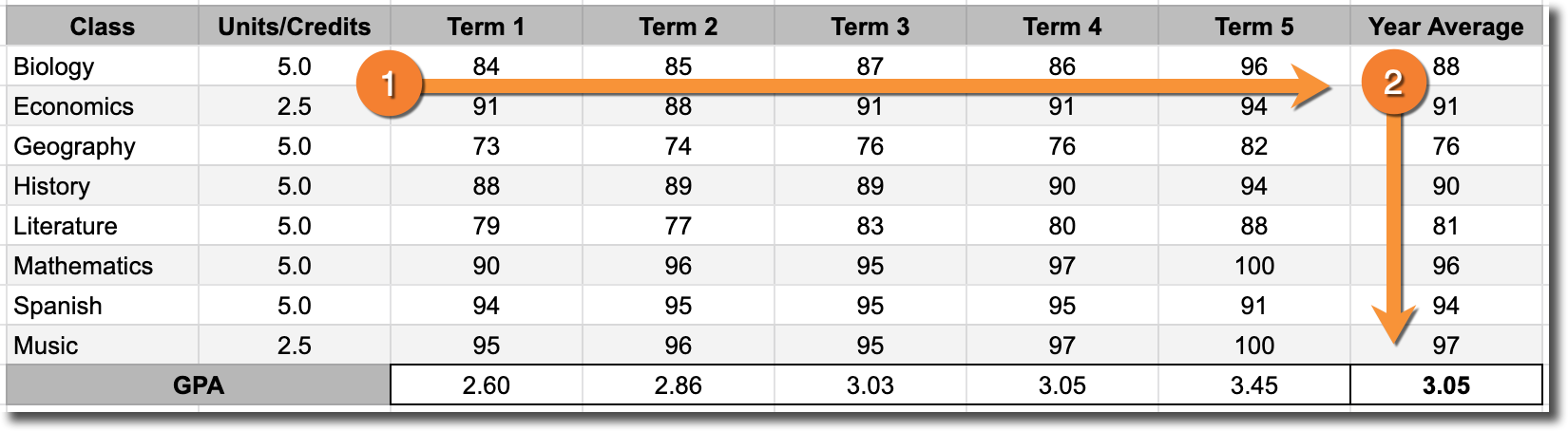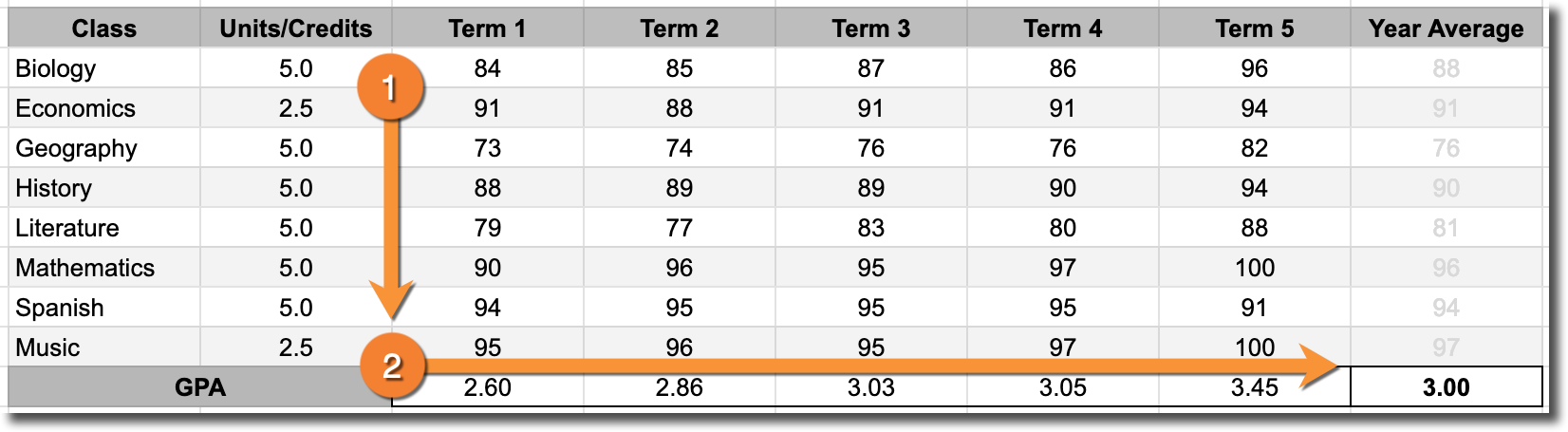On the GPA & Scores Report, it is possible for the same student to have different grade point averages (GPAs) and percent scores for the same academic term. The reason this possible is because Gradelink calculates GPA and Scores differently depending on which academic terms you select when generating the report.

Let's look at an example.This is the report card of a fictitious student that is enrolled in eight classes at a school where the academic year is divided into five terms. In Gradelink, that would look like this:When generating a GPA & Scores Report, if you only select the Parent Term ("School Year 2018-2019" in the image above) then Gradelink will calculate GPA by first averaging class grades together and then converting those averages into a GPA. Using this method, the student would have a GPA of 3.05.If you select all five of the 'Child Terms' instead of the Parent Term ("Term 1 2018-2019", "Term 2 2018-2019", etc.), then Gradelink will first calculate the GPA for each term and then average them together. Using this method, the student would have a GPA of 3.00.In addition to the use of slightly different methods when calculating GPA, there are other reasons why the scores might differ slightly. Consider this example:

Let's assume that "Student A" has an A+ in a class worth 5 units/credits and a B+ in a class worth 1 unit/credit. Let's also assume that "Student B" has a B+ in the same 5 unit class and an A+ in the same 1 unit class. If we assume that an A+ has a GPA value of 4.0 and a B+ has a GPA value of 3.0, then despite each student averaging a score of 90%, "Student A" will have a GPA of 3.83 and "Student B" will have a GPA of 3.16.